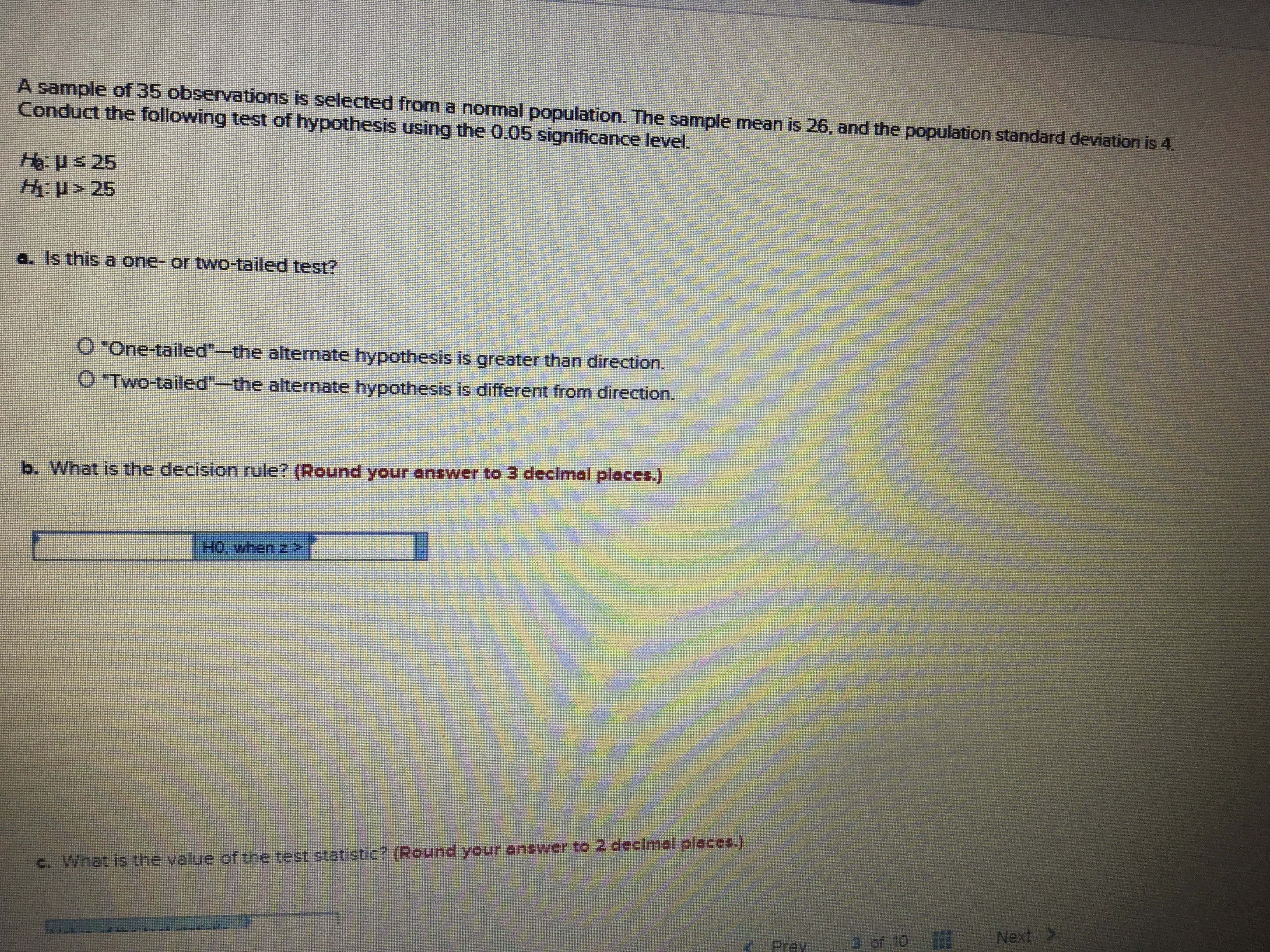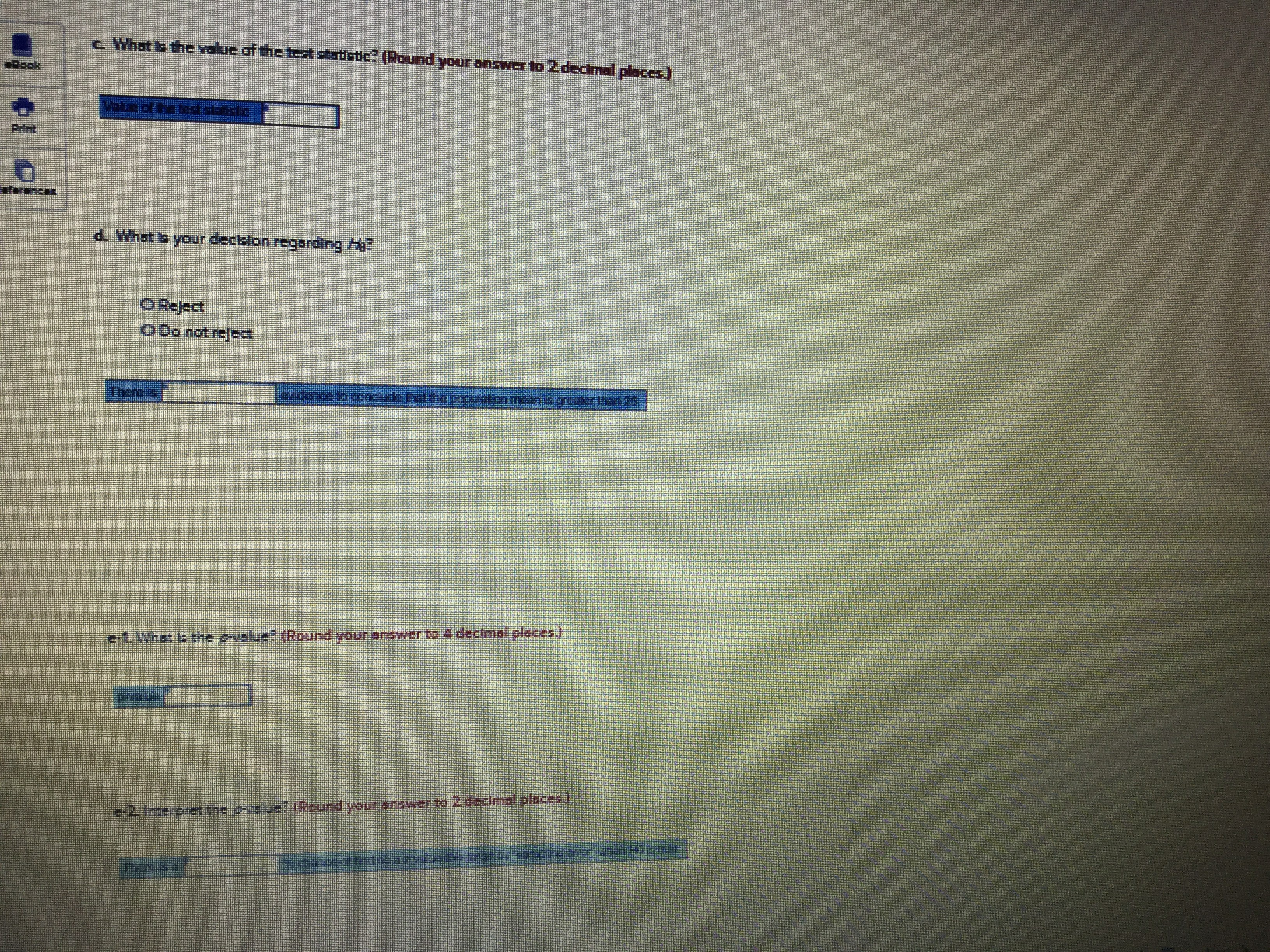# A sample of 35 observations is selected from a normal population. The sample mean is 26, and the population standard deviation is 4Conduct the following test of hypothesis using the O.05 significance level.H us 25: u> 25a. Is this a one- or two-tailed test?FOne-tailedthe alternate hypothesis is greater than direction.OTWO-tailed"-the altermate hypothesis is different from direction.b. What is the decision rule? (Round your answer to 3 declmal places.)Ho, when z>c. What is the value of the test statistic? (Round your enswer to 2 declmal places.)Next>of 10Prev c What s thelue of the tst sadc (Pound yourwer to 2 decmal places)**d. What s yourశండరion rogonongగేpofce ooDo not rejenenA dectms! places11e. 2.dండిగారి. O0ccs)Rsund yout:41282

Question
2 views

I. Would like solutions for all parts of the problemhelp_outlineImage TranscriptioncloseA sample of 35 observations is selected from a normal population. The sample mean is 26, and the population standard deviation is 4 Conduct the following test of hypothesis using the O.05 significance level. H us 25 : u> 25 a. Is this a one- or two-tailed test? FOne-tailed the alternate hypothesis is greater than direction. OTWO-tailed"-the altermate hypothesis is different from direction. b. What is the decision rule? (Round your answer to 3 declmal places.) Ho, when z> c. What is the value of the test statistic? (Round your enswer to 2 declmal places.) Next> of 10 Prev fullscreenhelp_outlineImage Transcriptionclosec What s thelue of the tst sadc (Pound your wer to 2 decmal places) ** d. What s your శండరion rogonongగే pofce o oDo not reje nen A dectms! places 11e. 2.dండిగారి. O0ccs) Rsund you t:41282 fullscreen
check_circle

Step 1

Given:

The provided sample mean is  = 26 and the known population standard deviation is σ = 4, and the sample size is n = 35.

The following null and alternative hypotheses need to be tested:

Step 2

a) This corresponds to a one-tailed test as alternate hypothesis is greater than direction. For which a z-test for one mean, with known population standard deviation and n>30 will be used.

b)

Decision rule:

Reject H0, when  z>1.645

Step 3

c)

Test statist...

### Want to see the full answer?

See Solution

#### Want to see this answer and more?

Solutions are written by subject experts who are available 24/7. Questions are typically answered within 1 hour.*

See Solution
*Response times may vary by subject and question.
Tagged in

### Hypothesis Testing